CDA数据科学研究院 CDA考试中心 企业服务 关于CDA

#全国校区## 用python转换PDF/Word/Excel/PPT等！2020-10-23Word转PDF

Word转PDF应该是最常见的需求了，毕竟使用PDF格式可以更方便展示文档，虽然在Word中可以直接导出为PDF格式，但是使用Python可以批量转换，更加高效。

`pip install docx2pdf`

```from docx2pdf import convert
convert("input.docx", "output.pdf")```

```#查找当前目录下的全部word文件
import os
import glob
from pathlib import Path

path = os.getcwd() + '/'
p = Path(path) #初始化构造Path对象
FileList=list(p.glob("**/*.docx")) ```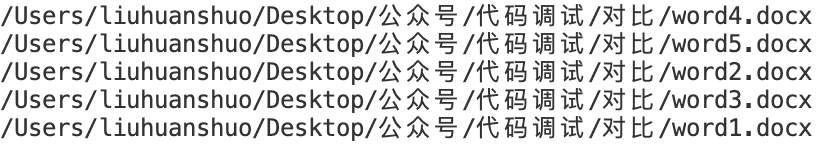```for file in FileList:
convert(file,f"{file}.pdf")```

Excel转PDF

Excel转PDF可能平时用的不多，但是作为Office全家桶中的重要工具，并且转换完的表格可以复制所以我们也讲一下。使用到的工具既不是常用的openpyxl也不是pandas，而是另一个专门用于处理PDF的库fpdf

```import pandas as pd
import numpy as np
df_1 = pd.DataFrame(np.random.randn(10, 2), columns=list('AB'))``````from fpdf import FPDF
pdf = FPDF()
pdf.set_xy(0, 0)
pdf.set_font('arial', 'B', 14)
pdf.cell(60)
pdf.cell(70, 10, 'Excel to PDF', 0, 2, 'C')
pdf.cell(-40)
pdf.cell(50, 10, 'Index Column', 1, 0, 'C')
pdf.cell(40, 10, 'A', 1, 0, 'C')
pdf.cell(40, 10, 'B', 1, 2, 'C')
pdf.cell(-90)
pdf.set_font('arial', '', 12)
for i in range(0, len(df_1)):
col_ind = str(i)
col_a = str(df_1.A.iloc[i])
col_b = str(df_1.B.iloc[i])
pdf.cell(50, 10, '%s' % (col_ind), 1, 0, 'C')
pdf.cell(40, 10, '%s' % (col_a), 0, 0, 'C')
pdf.cell(40, 10, '%s' % (col_b), 0, 2, 'C')
pdf.cell(-90)
pdf.output('Excel2PDF.pdf', 'F')```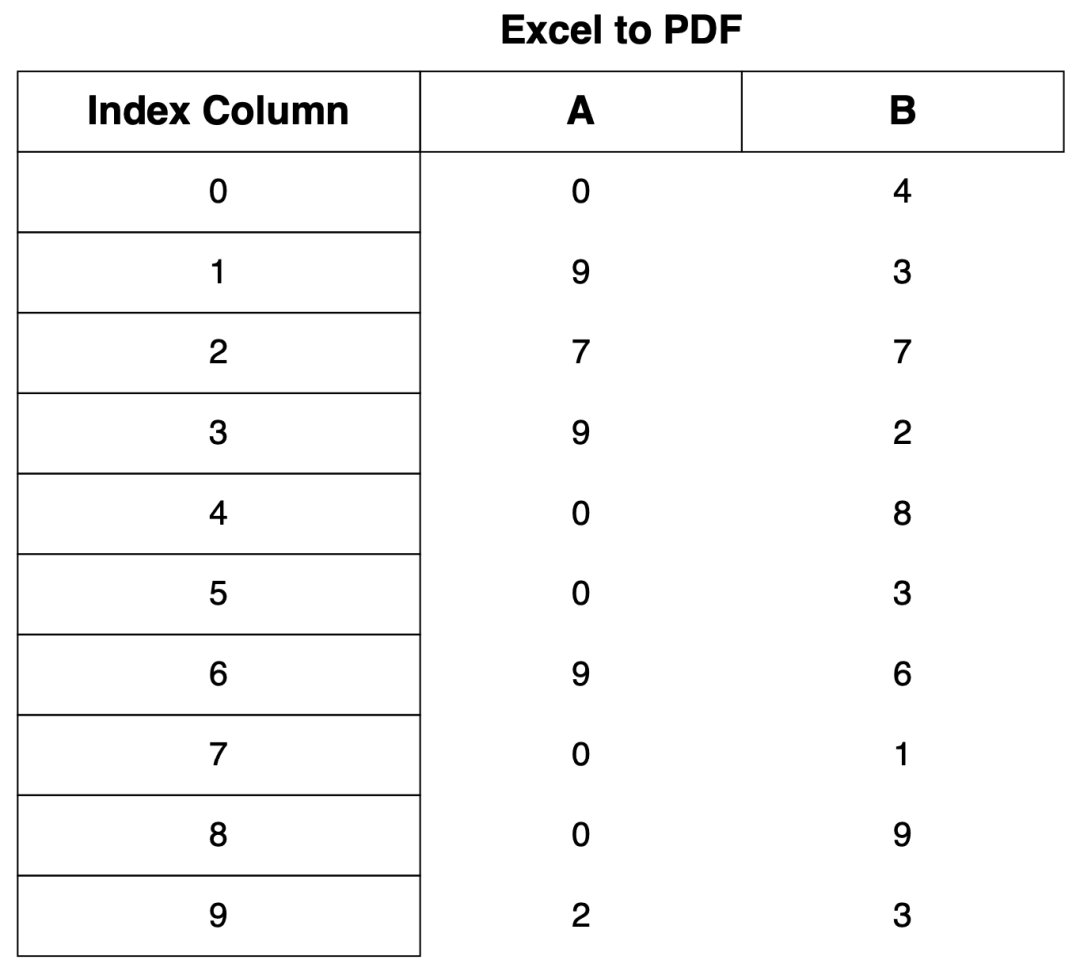，其实思路和openpyxl类似，遍历每一个单元格并写入数据，只不过现在是往PDF文件中写入。

PPT转PDF

```import sys
import os
import comtypes.client
#设置路径
input_file_path = sys.argv
output_file_path = sys.argv
input_file_path = os.path.abspath(input_file_path)
output_file_path = os.path.abspath(output_file_path)
#创建PDF
powerpoint = comtypes.client.CreateObject("Powerpoint.Application")
powerpoint.Visible = 1
slides = powerpoint.Presentations.Open(input_file_path)
#保存PDF
slides.SaveAs(output_file_path, 32)
slides.Close()```

md转pdf

```from markdown2pdf3 import *
convert_markdown_to_pdf('test.md') #你的markdown文件路径```

```import os
import glob
from pathlib import Path

path = os.getcwd() + '/'
p = Path(path) #初始化构造Path对象
FileList=list(p.glob("**/*.md")) ```

html转pdf

`https://wkhtmltopdf.org/downloads.html`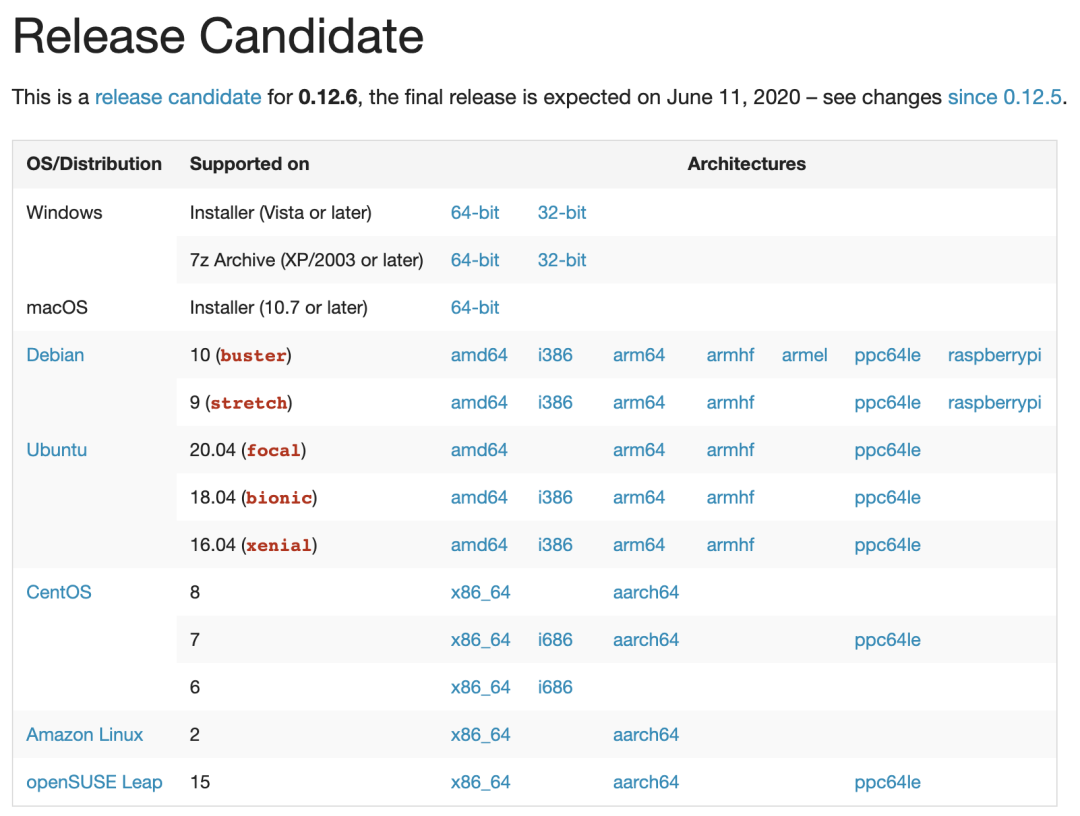`pip install pdfkit`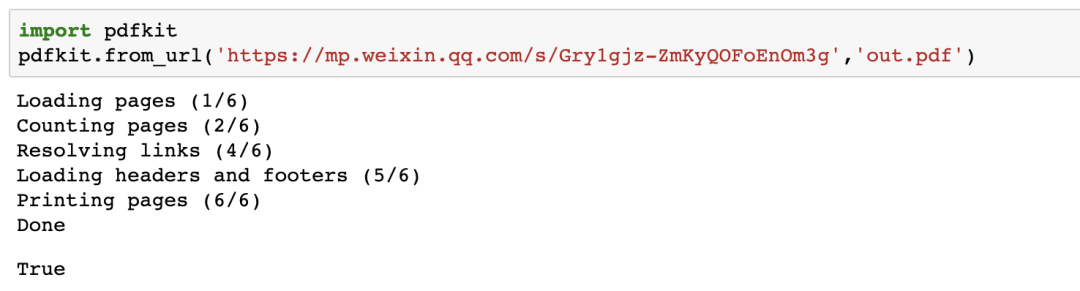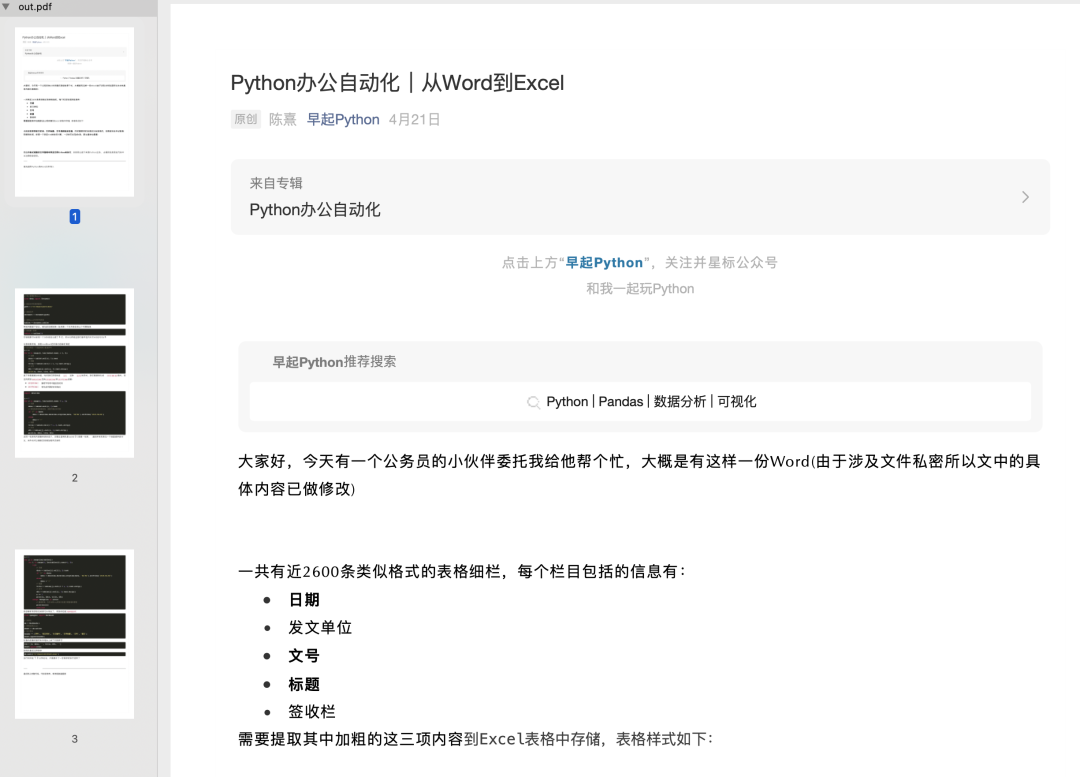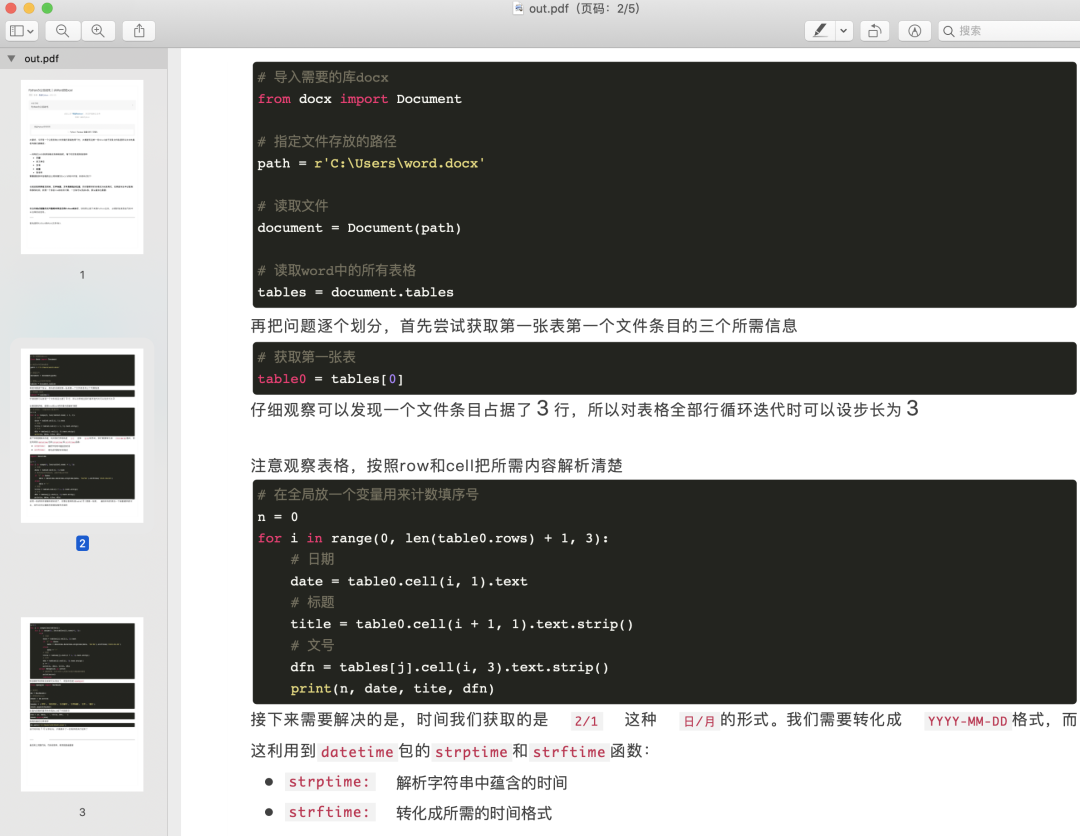——热门课程推荐：

OK